Home | | Maths 11th std | Exercise 7.5: Choose the correct answer

# Exercise 7.5: Choose the correct answer

Multiple choice questions with answers / choose the correct answer with answers - Maths Book back 1 mark questions and answers with solution for Exercise Problems - Mathematics : Matrices and Determinants

Matrices and Determinants (Mathematics)

## Choose the correct or the most suitable answer from the given four alternatives.

(1) If aij = 1/2 (3i − 2j ) and A = [a ij] 2×2 isAns: (2)

Solution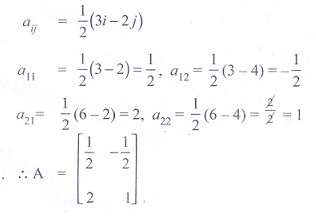(2) What must be the matrix X, ifAns: (1)

Solution(3) Which one of the following is not true about the matrix(1) a scalar matrix

(2) a diagonal matrix

(3) an upper triangular matrix

(4) a lower triangular matrix

Ans: (2)

(4) If A and B are two matrices such that A + B and AB are both defined, then

(1) A and B are two matrices not necessarily of same order

(2) A and B are square matrices of same order

(3) Number of columns of A is equal to the number of rows of B

(4) A = B.

Ans: (2)

Solution(5) If A =, then for what value of λ, A2 = O ?

(1) 0

(2) ±1

(3) - 1

(4) 1

Ans: (2)

Solution(6) Ifand ( A + B )2 = A2 + B2 then the values of a and b are

(1) a = 4, b = 1

(2) a = 1, b = 4

(3) a = 0, b = 4

(4) a = 2, b = 4

Ans: (2)

Solution(7) Ifis a matrix satisfying the equation  AAT = 9I, where I is 3 × 3 identity matrix, then the ordered pair (a, b) is equal to

(1) (2, - 1)

(2) (- 2, 1)

(3) (2, 1)

(4) (- 2, - 1)

Ans: (4)

Solution(8) If A is a square matrix, then which of the following is not symmetric?

(1) A + AT

(2) AAT

(3) AT

(4) A − AT

Ans: (4)

Solution(9) If A and B are symmetric matrices of order n, where ( A ≠ B), then

(1) A + B is skew-symmetric

(2) A + B is symmetric

(3) A + B is a diagonal matrix

(4) A + B is a zero matrix

Ans: (2)

Solution(10)  If A =and if xy = 1, then det ( AAT ) is equal to

(1) (a −1)2

(2) (a2 +1)2

(3) a2 −1

(4) (a2 −1)2

Ans: (4)

Solution(11) The value of x, for which the matrix A =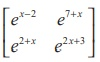is singular is

(1) 9

(2) 8

(3) 7

(4) 6

Ans: (2)

Solution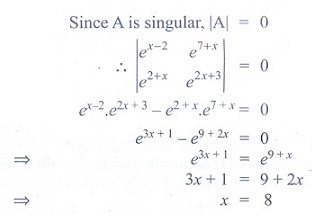(12) If the points (x, −2), (5, 2), (8,8) are collinear, then x is equal to

(1) - 3

(2) 1/3

(3) 1

(4) 3

Ans: (4)

Solution(13) If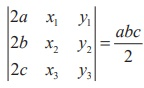= abc/2 ≠ 0, then the area of the triangle whose vertices areis

(1) 1/4

(2) 1/4abc

(3) 1/8

(4) 1/8 abc

Ans: (3)

Solution(14) If the square of the matrixis the unit matrix of order 2, then α, β and γ should satisfy the relation.

(1) 1+ α2 + βγ = 0

(2) 1− α2 − βγ = 0

(3) 1− α2 + βγ = 0

(4)  1+ α2 − βγ = 0

Ans: (2)

Solution(15) Ifis

(1) ∆

(2) k∆

(3) 3k∆

(4) k3

Ans: (4)

Solution(16) A root of the equation= 0 is

(1) 6

(2) 3

(3) 0

(4) - 6

Ans: (3)

Solution(17) The value of the determinant of A =is

(1) - 2abc

(2) abc

(3) 0

(4) a2 + b2 + c2

Ans: (3)

Solution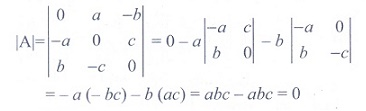(18) If  x1 , x2 , x3 as well as y1 , y 2 , y3 are in geometric progression with the same common ratio, then the points (x1 , y1 ), (x2 , y2 ), (x3 , y3) are

(1)  vertices of an equilateral triangle

(2)  vertices of a right angled triangle

(3)  vertices of a right angled isosceles triangle

(4) collinear

Ans: (4)

Solution(19)  Ifdenotes the greatest integer less than or equal to the real number under consideration and  −1 ≤ x < 0, 0 ≤ y < 1, 1 ≤ z < 2 , then the value of the determinantisAns: (1)

Solution(20) If a ≠ b, b, c satisfy= 0, then abc =

(1) a + b + c

(2) 0

(3) b3

(4) ab + bc

Ans: (3)

Solution(21) If, then B is given by

(1) B = 4A

(2) B = − 4A

(3) B = − A

(4) B = 6A

Ans: (2)

Solution(22) If A is skew-symmetric of order n and C is a column matrix of order n × 1, then CTAC is

(1) an identity matrix of order n

(2) an identity matrix of order 1

(3) a zero matrix of order 1

(4) an identity matrix of order 2

Ans: (3)

Solution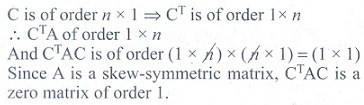(23) The matrix A satisfying the equationisAns: (3)

Solution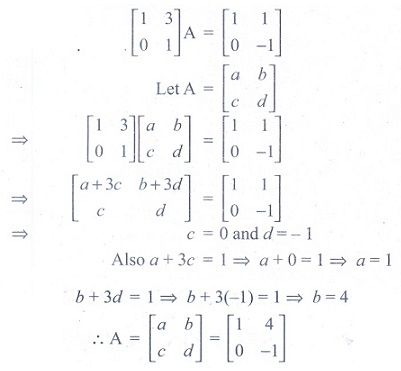(24) If A+ I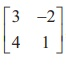, then ( A+I )( A-I ) is equal toAns: (1)

Solution(25)  Let A and B be two symmetric matrices of same order. Then which one of the following statement is not true?

(1) A + B is a symmetric matrix

(2) AB is a symmetric matrix

(3) AB = (BA)T

(4) ATB = ABT

Ans: (2)

SolutionTags : Matrices and Determinants | Mathematics , 11th Mathematics : UNIT 7 : Matrices and Determinants
Study Material, Lecturing Notes, Assignment, Reference, Wiki description explanation, brief detail
11th Mathematics : UNIT 7 : Matrices and Determinants : Exercise 7.5: Choose the correct answer | Matrices and Determinants | Mathematics